FRETBursts - μs-ALEX smFRET burst analysis¶

This notebook is part of a tutorial series for the FRETBursts burst analysis software.

In this notebook, we present a typical FRETBursts workflow for μs-ALEX smFRET burst analysis. Briefly, we show how to perform background estimation, burst search, burst selection, compute FRET histograms and ALEX histograms, do sub-population selection and finally, FRET efficiency fit.

If you are new to the notebook interface see First Steps before continuing.

Before running the notebook, you can click on menu Cell -> All Output -> Clear to clear all previous output. This will avoid mixing output from current execution and the previously saved one.

In :
from fretbursts import *
- Optimized (cython) burst search loaded.
- Optimized (cython) photon counting loaded.
--------------------------------------------------------------
You are running FRETBursts (version 0.6.4).

If you use this software please cite the following paper:

FRETBursts: An Open Source Toolkit for Analysis of Freely-Diffusing Single-Molecule FRET
Ingargiola et al. (2016). http://dx.doi.org/10.1371/journal.pone.0160716

--------------------------------------------------------------
Thanks in advance for remembering to cite FRETBursts in publications or presentations!

Note that FRETBursts version string tells you the exact FRETBursts version (and revision) in use. Storing the version in the notebook helps with reproducibility and tracking software regressions.

Next, we initialize the default plot style for the notebook (under the hood it uses seaborn):

In :
sns = init_notebook()

Note that the previous command has no output. Finally, we print the version of some dependencies:

In :
import lmfit; lmfit.__version__
Out:
'0.9.7'
In :
import phconvert; phconvert.__version__
Out:
'0.7.3'

The full list of smFRET measurements used in the FRETBursts tutorials can be found on Figshare.

In :
url = 'http://files.figshare.com/2182601/0023uLRpitc_NTP_20dT_0.5GndCl.hdf5'
You can change the url variable above to download your own data file. This is useful if you are executing FRETBursts online and you want to use your own data file. See First Steps.

Here, we download the data file and put it in a folder named data, inside the notebook folder:

In :
URL:  http://files.figshare.com/2182601/0023uLRpitc_NTP_20dT_0.5GndCl.hdf5
File: 0023uLRpitc_NTP_20dT_0.5GndCl.hdf5

NOTE: If you modified the url variable providing an invalid URL the previous command will fail. In this case, edit the cell containing the url and re-try the download.

Selecting the data file¶

Use one of the following 2 methods to select a data file.

Option 1: Paste the file-name¶

Here, we can directly define the file name to be loaded:

In :
filename = "./data/0023uLRpitc_NTP_20dT_0.5GndCl.hdf5"
filename
Out:
'./data/0023uLRpitc_NTP_20dT_0.5GndCl.hdf5'

Now filename contains the path of the file you just selected. Run again the previous cell to select a new file. In a following cell we will check if the file actually exists.

When running the notebook online and using your own data file, make sure to modify the previous cell.
See First Steps.

Option 2: Use an "Open File" dialog¶

Alternatively, you can select a data file with an "Open File" windows. Note that, since this only works in a local installation, the next commands are commented (so nothing will happen when running the cell).

If you want to try the File Dialog, you need to remove the # signs:

In :
# filename = OpenFileDialog()
# filename

Check that the data file exists¶

Let's check that the file exists:

In :
import os
if os.path.isfile(filename):
print("Perfect, file found!")
else:
Perfect, file found!

We can finally load the data and store it in a variable called d:

In :

If you don't get any message, the file is loaded successfully.

We can also set the 3 correction coefficients:

• leakage or bleed-through: leakage
• direct excitation: dir_ex (ALEX-only)
• gamma-factor gamma
In :
d.leakage = 0.11
d.dir_ex = 0.04
d.gamma = 1.

NOTE: at any later moment, after burst search, a simple reassignment of these coefficient will update the burst data with the new correction values.

μs-ALEX parameters¶

At this point, timestamps and detectors numbers are contained in the ph_times_t and det_t attributes of d. Let's print them:

In :
d.ph_times_t, d.det_t
Out:
([array([     146847,      188045,      294124, ..., 47999863658,
47999877783, 47999955353])],
[array([0, 1, 1, ..., 1, 1, 0], dtype=uint32)])

We need to define some ALEX parameters:

In :
alex_period = 4000,
offset = 700,
D_ON = (2180, 3900),
A_ON = (200, 1800))

Here the parameters are:

• det_donor_accept: donor and acceptor channels
• alex_period: length of excitation period (in timestamps units)
• D_ON and A_ON: donor and acceptor excitation windows
• offset: the offset between the start of alternation and start of timestamping (see also Definition of alternation periods).

To check that the above parameters are correct, we need to plot the histogram of timestamps (modulo the alternation period) and superimpose the two excitation period definitions to it:

In :
bpl.plot_alternation_hist(d)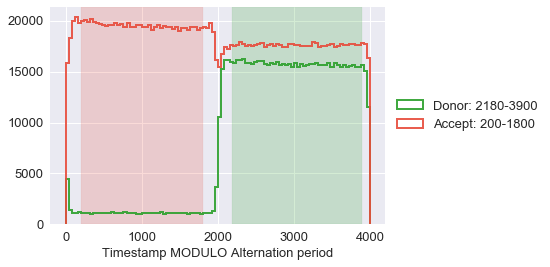If the previous alternation histogram looks correct, the corresponding definitions of the excitation periods can be applied to the data using the following command:

In :
# Total photons (after ALEX selection):     2,259,522
#  D  photons in D+A excitation periods:      721,537
#  A  photons in D+A excitation periods:    1,537,985
# D+A photons in  D  excitation period:     1,434,842
# D+A photons in  A  excitation period:       824,680

If the previous histogram does not look right, the parameters in the d.add(...) cell can be modified and checked by running the histogram plot cell until everything looks fine. Don't forget to apply the parameters with loader.usalex_apply_period(d) as a last step.

NOTE: After applying the ALEX parameters a new array of timestamps containing only photons inside the excitation periods is created (name d.ph_times_m). To save memory, by default, the old timestamps array (d.ph_times_t) is deleted. Therefore, in the following, when we talk about all-photon selection we always refer to all photons inside both excitation periods.

Measurement infos¶

The entire measurement data is now stored in the variable d. Printing it will give a compact representation containing the file-name and additional parameters:

In :
d
Out:
data_0023uLRpitc_NTP_20dT_0.5GndCl G1.000 Lk11.000 dir4.0

To check the measurement duration (in seconds) run:

In :
d.time_max
Out:
599.99943316249994

Plotting basics¶

In this section basic concepts of plotting with FRETBursts using the timetrace plot as an example.

To plot a timetrace of the measurement we use:

In :
dplot(d, timetrace);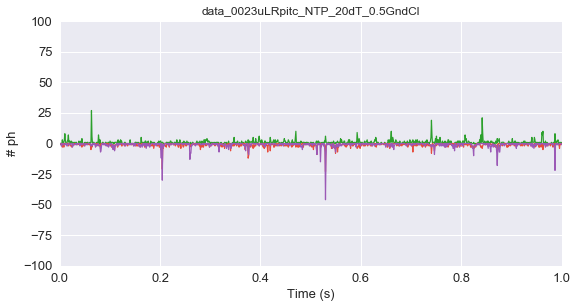Here, dplot is a generic wrapper (the same for all plots) that takes care of setting up the figure, title and axis (in the multispot case dplot creates multi-panel plot). The second argument, timetrace, is the actual plot function. All the eventual additional arguments passed to dplot are, in turn, passed to the plot function (e.g. timetrace).

If we look at the documentation for timetrace function we notice that it accepts a long list of arguments. In python, when an argument is not specified, it will take the default value specified in the function definition (see previus link).

As an example, to change the bin size (i.e. duration) of the timetrace histogram, we can look up in the timetrace documentation and find that the argument we need to modify is binwidth (we can also see that the default value is 0.001 seconds). We can then re-plot the timetrace using a bin of 0.5 ms:

In :
dplot(d, timetrace, binwidth=0.5e-3);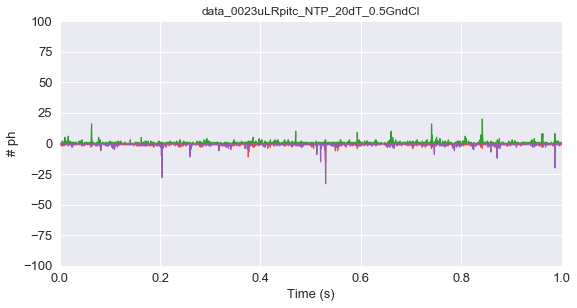The timetrace is computed between tmin and tmax (by default 0 and 200s), but as you can see is displayed only between 0 an 1 second, just because these are the default x-axis limits. The axis limits can be changes by using the standard matplotlib command plt.xlim(). On the other hand, to change the range where the timetrace is computed, we pass the additional arguments tmin and tmax as follows:

In :
dplot(d, timetrace, binwidth=0.5e-3, tmin=50, tmax=150)
plt.xlim(51, 52);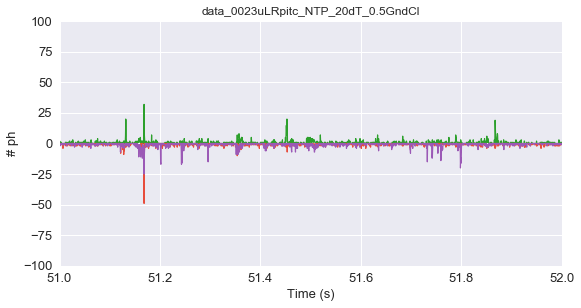When using FRETBursts in a notebook, all plots are static by default. This is because we use the so called inline backend of matplotlib. If you want to manipulate figures interactively, you can switch to the interactive notebook backend with:

%matplotlib notebook

to go back to inline use:

%matplotlib inline

NOTE: Currently, the notebook backend is incompatible with the QT backend. If in a session you activate the notebook backend, then switching to the QT backend requires restarting the notebook. Conversely, you can switch between inline and notebook or between inline and qt4 backends in the same session wihtou issues.

Background estimation¶

As a first step of the analysis, we need to estimate the background. The assumption is that the background is a Poisson process and therefore the corresponding inter photon delays are exponentially distributed. Since the background can change during the measurement, a new estimation is computed every time_s seconds (this time is called the background period).

The inter photon delay distribution contains both single-molecule signal and background, the latter being the only one we are interested in and the former being in general much shorter. Therefore, a threshold is needed to discriminate between the exponential tail and the single-molecule peak.

Choosing a threshold and fitting the exponential tail are two different problems. FRETBursts provides several ways to specify the minimum threshold and different functions to fit the exponential tail.

We will go over three different methods in increasing order of complexity (and recommendability)

Single threshold¶

Let start with a standard Maximum Likelihood (ML) background fit with a minimum tail threshold of 500μs:

In :
d.calc_bg(bg.exp_fit, time_s=1000, tail_min_us=500)
- Calculating BG rates ... [DONE]

We can look at how the fit looks with:

In :
dplot(d, hist_bg, show_fit=True)
Out:
<matplotlib.axes._subplots.AxesSubplot at 0x127a1b6d8>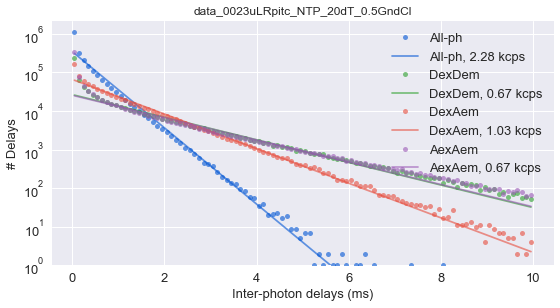Note that the fits are not very good. This is understandable because we used a single threshold for all the photon streams, each one having a quite different background.

Multiple thresholds¶

To improve the fit, we can try specifying a threshold for each channel. This method is bit ad-hoc but it may work well when the thresholds are properly choosen.

In :
d.calc_bg(bg.exp_fit, time_s=1000, tail_min_us=(800, 4000, 1500, 1000, 3000))
- Calculating BG rates ... [DONE]
In :
dplot(d, hist_bg, show_fit=True);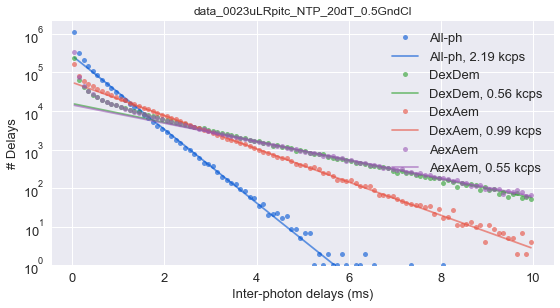For ALEX measurements, the tuple passed to tail_min_us in order to define the thresholds needs to contain
5 values corresponding the 5 distinct photon streams (the all-photon stream

• the 4 base alternation streams). The order of these 5 values need to match the order of photon streams in the Data.ph_streams attribute:
In :
d.ph_streams
Out:
[Ph_sel(Dex='DAem', Aex='DAem'),
Ph_sel(Dex='Dem', Aex=None),
Ph_sel(Dex='Aem', Aex=None),
Ph_sel(Dex=None, Aex='Dem'),
Ph_sel(Dex=None, Aex='Aem')]

Automatic threshold¶

Finally, is possible to let FRETBursts infer the threshold automatically with:

In :
d.calc_bg(bg.exp_fit, time_s=1000, tail_min_us='auto', F_bg=1.7)
- Calculating BG rates ... [DONE]

Which results in the following fit plot:

In :
dplot(d, hist_bg, show_fit=True);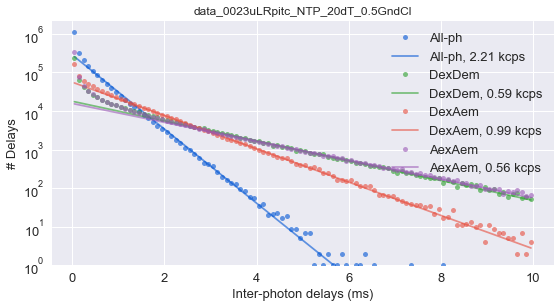Under the hood, this method estimates the threshold automatically according to this formula:

threshold_auto = F_bg / coarse_background_rate

where F_bg is the fit function input argument (by default 1.7) and coarse_background_rate is an initial background estimation performed with fixed threshold. This method is concemptually an iterative method to compute the threshold that is stopped after the second iteration (this is usually more than enough for accurate estimates).

Of the three methods here described, the latter is the recommended one since it works well and without user intervention in a wide range of experimental conditions.

Background timetrace¶

It is a good practice to monitor background rates as a function of time. Here, we compute background in adjacent 30s windows (called background periods) and plot the estimated rates as a function of time.

In :
d.calc_bg(bg.exp_fit, time_s=30, tail_min_us='auto', F_bg=1.7)
- Calculating BG rates ... [DONE]
In :
dplot(d, timetrace_bg)
Out:
<matplotlib.axes._subplots.AxesSubplot at 0x127a2c7f0>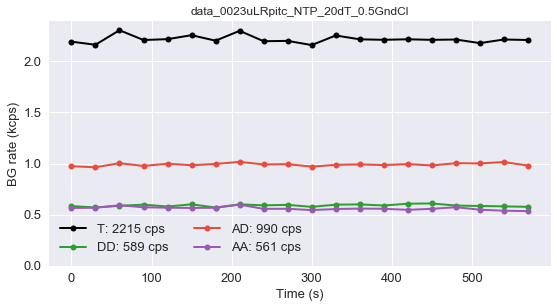Getting the background rates¶

The background rates are stored in Data() attribute bg, a dict with photon streams (Ph_sel objects) as key. Each item in the dict contains a list of fitted background rates for each channel and period.

In :
d.bg
Out:
{Ph_sel(Dex='DAem', Aex='DAem'): [array([ 2192.33771456,  2162.14083635,  2304.31199333,  2208.57734993,
2216.88852285,  2255.68759074,  2202.02910176,  2298.45997825,
2196.7975027 ,  2199.98590374,  2159.05120194,  2252.82753492,
2215.34278931,  2210.60163812,  2215.6184806 ,  2210.1510978 ,
2213.32385234,  2178.18199206,  2213.60242855,  2208.64479515])],
Ph_sel(Dex='Dem', Aex=None): [array([ 584.18970691,  570.58641253,  586.80564353,  598.45561342,
579.68811571,  601.34029113,  569.74563784,  601.248969  ,
591.47186394,  596.06342699,  576.64661876,  598.0513001 ,
600.65952237,  589.70963389,  607.49394193,  610.00901454,
589.13559128,  585.2150928 ,  581.32356205,  576.791585  ])],
Ph_sel(Dex='Aem', Aex=None): [array([  973.96356856,   963.80151895,  1003.656506  ,   976.56430321,
998.56747257,   983.73415654,   996.47590969,  1016.73812567,
991.14920045,   994.47939727,   970.14966698,   987.63026179,
991.74747802,   985.56352863,   994.77741329,   981.68939719,
1003.74305566,  1001.2290634 ,  1015.02463123,   980.3738204 ])],
Ph_sel(Dex=None, Aex='Dem'): [array([ 81.25392506,  77.34935176,  70.85741407,  73.75133485,
76.24222792,  77.82545767,  73.1705312 ,  78.46092124,
70.92503618,  71.6808552 ,  75.55277658,  77.84662415,
74.29129798,  69.49896567,  74.36290656,  77.00695872,
74.03801857,  72.81002019,  73.92609799,  74.57414299])],
Ph_sel(Dex=None, Aex='Aem'): [array([ 565.41275495,  567.69856299,  592.02680332,  572.78126164,
568.49858848,  565.73474853,  567.51131955,  598.30138811,
556.9935006 ,  557.87730382,  544.68106085,  555.38189993,
559.75536058,  557.55247497,  547.56454569,  557.25229028,
572.92889217,  548.83714375,  538.59524696,  535.0871576 ])]}

We can also get the average background for each channel:

In :
d.bg_mean
Out:
{Ph_sel(Dex='DAem', Aex='DAem'): [2215.7281152497922],
Ph_sel(Dex='Dem', Aex=None): [589.73157718553762],
Ph_sel(Dex='Aem', Aex=None): [990.55292377373189],
Ph_sel(Dex=None, Aex='Dem'): [74.771243227050803],
Ph_sel(Dex=None, Aex='Aem'): [561.52361523921024]}

Burst analysis¶

The first step of burst analysis is the burst search.

We will use the sliding-window algorithm on all photons. Note that "all photons", as mentioned before, means all photons selected in the alternation histogram. An important variation compared to the classical sliding-windows is that the threshold-rate for burst start is computed as a function of the background and changes when the background changes during the measurement.

To perform a burst search evaluating the photon rate with 10 photons (m=10), and selecting a minimum rate 6 times larger than the background rate (F=6) calculated with all photons (default):

In :
d.burst_search(L=10, m=10, F=6)
- Performing burst search (verbose=False) ...[DONE]
- Calculating burst periods ...[DONE]
- Counting D and A ph and calculating FRET ...
- Applying background correction.
- Applying leakage correction.
- Applying direct excitation correction.
[DONE Counting D/A]

The previous command performs the burst search, corrects the bursts sizes for background, spectral leakage and direct excitation, and computes $\gamma$-corrected FRET and Stoichiometry.

See the burst_search documentation for more details.

We can plot the resulting FRET histogram using the following command:

In :
dplot(d, hist_fret);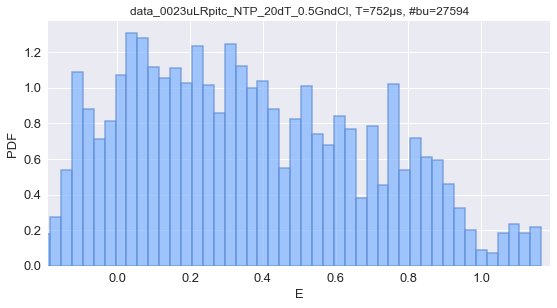All pre-defined plots follow this pattern: call the generic dplot() function, passing 2 parameters:

• the measurement data (d in this case)
• the plot function (hist_fret)

In some case we can add other optional parameters to tweak the plot.

All plot functions start with hist_ for histograms, scatter_ for scatter-plots or timetrace_ for plots as a function of measurement time. You can use autocompletion to find all plot function or you can look in bursts_plot.py where all plot functions are defined.

Instead of hist_fret we can use hist_fret_kde to add a KDE overlay. Also, we can plot a weighted histogram by passing an additional parameter weights:

In :
dplot(d, hist_fret, show_kde=True);
dplot(d, hist_fret, show_kde=True, weights='size');
- Overwriting the old E_fitter object with the new weights.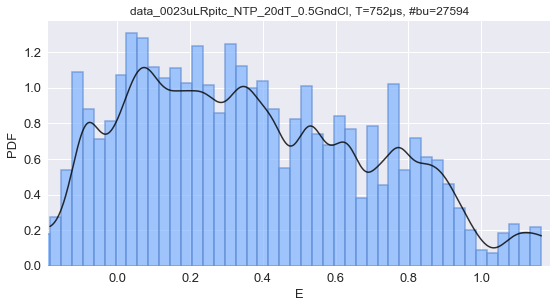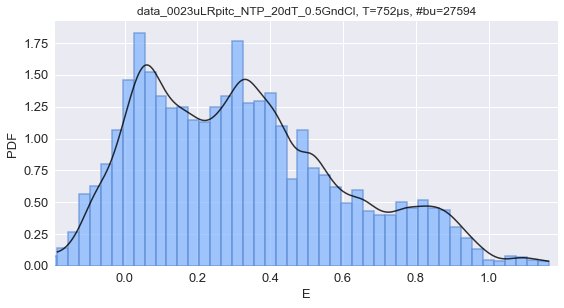You can experiment with different weighting schema (for all supported weights see get_weigths() function in fret_fit.py).

Burst selection¶

When we performed the burst search, we specified L=10 without explaining what this parameter means. L is traditionally the minimum size (number of photons) for a burst: smaller bursts will be rejected. By setting L=m (10 in this case) we are deciding to not discard any burst (because the smallest detected burst has at least m counts).

Selecting the bursts in a second step, by applying a minimum burst size criterion, results in a more accurate and unbiased selection.

For example, we can select bursts with more than 30 photons (after background, gamma, leakage and direct excitation corrections) and store the result in a new Data() variable ds:

In :
ds = d.select_bursts(select_bursts.size, th1=30)

By defaults the burst size includes donor and acceptor photons during donor excitation. To add acceptor photons during acceptor excitation (naa), we add the parameter add_naa=True:

In :

Similar to plot functions, all selection functions are defined in select_bursts.py and you can access them by typing select_bursts. and using the TAB key for autocompletion.

To replot the FRET histogram after selection (note that now we are passing ds to the plot function):

In :
dplot(ds, hist_fret);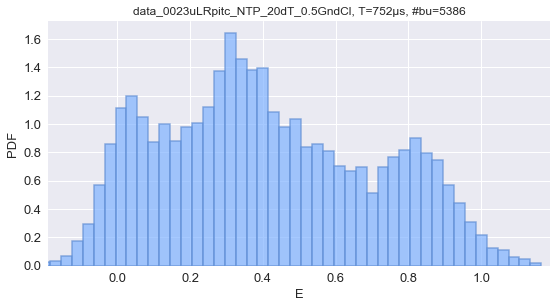Note how the histogram exhibits much more clearly defined peaks after burst selection.

Histogram fitting and plotting style¶

Under the hood the previous hist_fret plot creates a MultiFitter object for $E$ values. This object, stored as ds.E_fitter, operates on multi-channel data and computes the histogram, KDE and can fit the histogram with a model (lmfit.Model).

Now, just for illustration purposes, we fit the previous histogram with 3 Gaussians, using the already created ds.E_fitter object:

In :
ds.E_fitter.fit_histogram(mfit.factory_three_gaussians(), verbose=False)
In :
dplot(ds, hist_fret, show_model=True);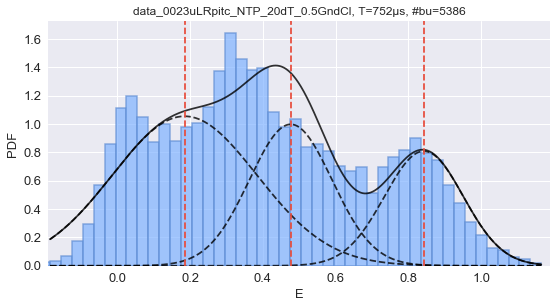The bin width can be changed with binwidth argument. Alternatively, an arbitrary array of bin edges can be passed in bins (overriding binwidth).

We can customize the appearance of this plot (type hist_fret? for the complete set of arguments).

For example to change from a bar plot to a line-plot we use the hist_style argument:

In :
dplot(ds, hist_fret, show_model=True, hist_style='line')
Out:
<matplotlib.axes._subplots.AxesSubplot at 0x127d5fef0>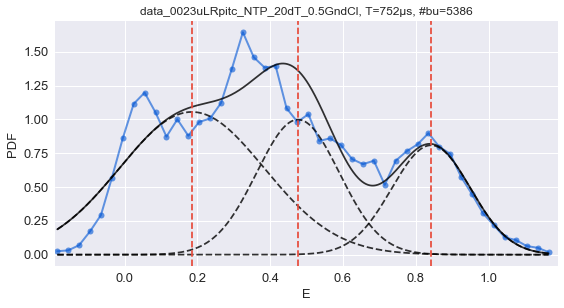We can customize the line-plot, bar-plot, the model plot and the KDE plot by passing dictionaries with matplotlib style. The name of the arguments are:

• hist_plot_style: style for the histogram line-plot
• hist_bar_style: style for the histogram bar-plot
• model_plot_style: style for the model plot
• kde_plot_style: style for the KDE plot

As an example:

In :
dplot(ds, hist_fret, show_model=True, hist_style='bar', show_kde=True,
kde_plot_style = dict(linewidth=5, color='orange', alpha=0.6),
hist_plot_style = dict(linewidth=3, markersize=8, color='b', alpha=0.6))
plt.legend();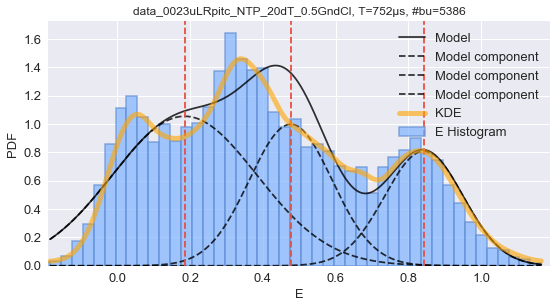Other plots¶

Similarly, we can plot the burst size using all photons (type hist_size? to learn about all plot options):

In :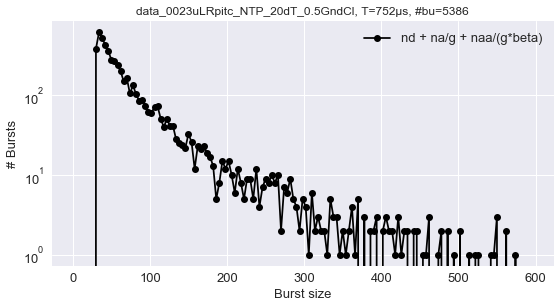Or plot the burst size histogram for the different components:

In :
dplot(ds, hist_size_all);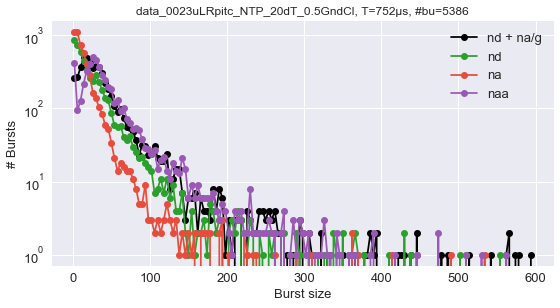NOTE: The previous plot may generate a benign warning due to the presence of zeroes when switching to log scale. Just ignore it.

A scatterplot of Size vs FRET is created by:

In :
dplot(ds, scatter_fret_nd_na)
xlim(-1, 2)
Out:
(-1, 2)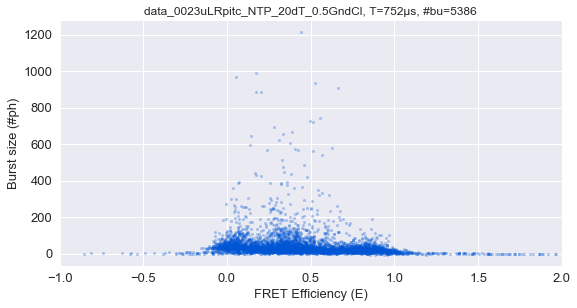Study of different populations¶

Removing multi-mers¶

We can further select only bursts smaller than 300 photons to get rid of multi-molecule events:

In :
ds2 = ds.select_bursts(select_bursts.size, th2=300)

and superimpose the two histograms before and after selection to see the difference:

In :
ax = dplot(ds2, hist_fret, hist_style='bar', show_kde=True,
hist_bar_style = dict(facecolor='r', alpha=0.5,
label='Hist. no large bursts'),
kde_plot_style = dict(lw=3, color='m',
label='KDE no large bursts'))
dplot(ds, hist_fret, ax=ax, hist_style='bar', show_kde=True,
hist_bar_style = dict(label='Hist. with large bursts'),
kde_plot_style = dict(lw=3, label='KDE with large bursts'))
plt.legend();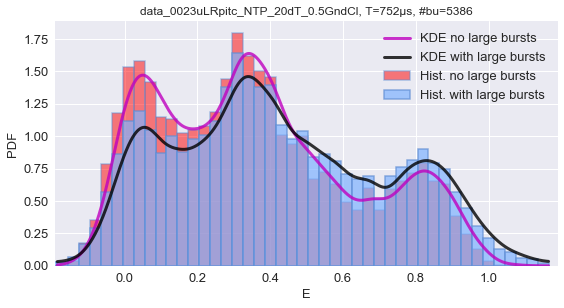NOTE: It is not necessarily true that bursts with more that 300 photons represents multiple molecules. To asses the valididty of this assumption it can be useful to plot the peak count rates in each burst. See hist_burst_phrate for this kind of plot.

Fit and plot peak positions¶

We can find the KDE peak position in a range (let say 0.2 ... 0.6):

In :
ds.E_fitter.find_kde_max(np.r_[0:1:0.0002], xmin=0.2, xmax=0.6)

and plot it with show_kde_peak=True, we also use show_fit_value=True to show a box with the fitted value:

In :
dplot(ds, hist_fret, hist_style='line',
show_fit_value=True,
show_kde=True, show_kde_peak=True);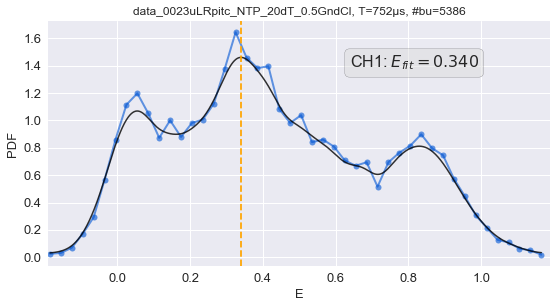Instead of using the KDE, we can use the peak position as fitted from a gaussian model.

In :
ds.E_fitter.fit_histogram(mfit.factory_three_gaussians(), verbose=False)

To select which peak to show we use fit_from='p1_center':

In :
dplot(ds, hist_fret, hist_style='line',
show_fit_value=True, fit_from='p2_center',
show_model=True);